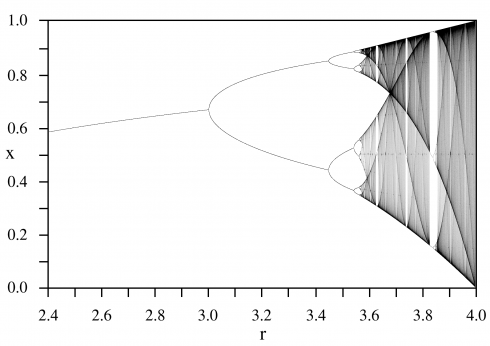# From Chaotic behaviour to Random Numbers

Fig.1 - The Relative number of appearances of the word "random" in Google Books database.

## What is randomness?

The definition of "random" given by the Collins dictionary is:

"If you describe events as random, you mean that they do not seem to follow a definite plan or pattern."

The word "seem" in this definition for our purposes will differentiate pseudo-random (do not seem) from the random (do not) definition. The word "seem" has a crucial implication in our vision of nature. In fact, by adopting this definition, If the world followed classical physics, randomness would not exist. The intrinsically deterministic and time-symmetric principles of the classical physic prevent the arising of truly random processes. True physical randomness is conceived uniquely by the quantum theory (in its probabilistic interpretation). Nevertheless, the extraordinarily high number of degrees of freedom of our universe and the non-linearity of the governing laws allows the emergence of the (pseudo) random processes even when the quantum phenomena are irrelevant.

Summing up:

1. Quantum-like processes are theoretically accepted to be subject to real randomness $\Rightarrow$ non-deterministic behaviour (click here to see how to create random numbers in this way);

2. Deterministic calculations cannot yield to random numbers because by definition they follow a rule;

3. Non-linear deterministic maps can yield effectively unpredictable sequences of pseudo-random numbers $\Rightarrow$ deterministic chaotic behaviour (pseudorandom-generators follow this way).

## The Logistic Map - deterministic chaos

A logistic map is one of the simplest non-linear discrete dynamic system representation. A continuous dynamical systems is a system that can be described by an equation of the type: $$\frac{{\rm d} s} {{\rm d} t} = f(s)$$ Where s is the state variable and t is the time. We can write the discrete time equivalent system as: $$s_{t+1}=f(s_t)$$ The easiest possible time-discrete dynamic equation is the linear map $$s_{t+1}=\gamma s_t$$ The logistic map is a non-linear time-discrete map defined as: $$s_{t+1} = F\left(x_s\right) = \gamma s_t \cdot (1-s_t)$$ as you can see the state of the system s at the time step $t+1$ depends in a non linear way on the system state at time $t$. Depending on the value of $\gamma$ the behaviour of the system changes:

• If $0 < \gamma < 3.5$ the system is bounded in the interval $[0,1]$
• if $\gamma < 3$ the system is stable and has a fixed point $S = \dfrac{\gamma-1}{\gamma}$
• if $3 < \gamma < 1 + \sqrt{6}$ the system starts to oscillate between two fixed points $\Rightarrow$  bifurcation $$S_{1,2} = \left[ \gamma +1 \pm ((\gamma +1)(\gamma -3))^{1/2} \right] / 2\gamma$$ where $S_1 = F(S_2) \quad S_2 = F(S_1)$
• at $\gamma > 1+\sqrt{6}$ there is a so called period doubling and the $s_t$ flips around between 4 points.
• $3 < \gamma < 3.57$ infinite number of period doubling occurs as $\gamma \uparrow$
• $a > 3.57$ the behavior becomes aperiodic and chaotic, nevertheless there still exist islands of tranquility in the sea of chaos in which the periodic behavior is recovered.Fig. - Bifurcation diagram for the logistic map. The attractor for any value of the parameter r is shown on the vertical line at that r(Wikipedia)

### Exercise

• In your opinion what is the difference between a chaotic and a random behaviour?
• Implement the logistic map in c++ and, using high values of gamma, compare the generated numbers with the random number generators that you implemented in TP1.
• Discuss the stability of 2-flip points condition.
• Do you think that the output of the logistic map can be seen as a random number generator or there are some missing proprieties?

## random number generators

The random number generator can be of two types:

1. hardware random-number generators;
2. pseudo-random number generators (PRNG).

The first class takes advantage of quantum processes to generate real random number sequences. The second class instead, consist of algorithms that starting from an initial value (seed) generate a sequence of numbers that follow a chaotic behaviour, but that must have some other important features:

• uniformly distributed $\Rightarrow$ from a uniformly distributed random sequence is easy to do a mapping to a non-uniform distribution of random numbers
• the generated numbers should be (almost) uncorrelated $\Rightarrow$ the sequence should pass all the random test (these tests are usually derived from the $\chi^2$ Pearson test);
• long period: with finite precision arithmetic a sequence must repeat itself, its period must be much longer of the random numbers needed by the application
• insensitivity to seed: the above properties should not depend on the initial seed.